Frequency Response

# Frequency Response Notes | Study Analog Circuits - Electronics and Communication Engineering (ECE)

## Document Description: Frequency Response for Electronics and Communication Engineering (ECE) 2022 is part of Frequency Response for Analog Circuits preparation. The notes and questions for Frequency Response have been prepared according to the Electronics and Communication Engineering (ECE) exam syllabus. Information about Frequency Response covers topics like Frequency Response of Amplifiers and Frequency Response Example, for Electronics and Communication Engineering (ECE) 2022 Exam. Find important definitions, questions, notes, meanings, examples, exercises and tests below for Frequency Response.

Introduction of Frequency Response in English is available as part of our Analog Circuits for Electronics and Communication Engineering (ECE) & Frequency Response in Hindi for Analog Circuits course. Download more important topics related with Frequency Response, notes, lectures and mock test series for Electronics and Communication Engineering (ECE) Exam by signing up for free. Electronics and Communication Engineering (ECE): Frequency Response Notes | Study Analog Circuits - Electronics and Communication Engineering (ECE)
 1 Crore+ students have signed up on EduRev. Have you?

Frequency Response of Amplifiers

➤ Introduction

• Amplifiers do not have the same gain at all frequencies.
• An amplifier designed for radio frequencies will amplify a band of frequencies above about 100kHz but will not amplify the lower frequency audio signals.
• In each case the amplifier has a particular frequency response, being a band of frequencies where it provides adequate amplification, and excluding frequencies above and below this band, where the amplification is less than adequate.

➤ Hybrid Equivalent Circuit for a Transistor

• Any single stage transistor amplifier, whether CB, CE or CC may be put in a standard form for small signal analysis so as to be able to identify Vg, Rg, and RL as well as the transistor’s input and output terminals.
• The hybrid equivalent circuit for a transistor consists of a Thevenin equivalent circuit at the input and a Norton equivalent circuit at the output.
• The equations relating the input and output voltages and currents in a transistors small signal equivalent circuit are given by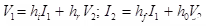• h= Input impedancehr = Reverse voltage ratio
• hf = Forward current transfer ratio
• where, hi, hr, hf and h0 are the h-parameters and have a second subscript of b, e or c according to the amplifier in question.
• The h-parameters may be obtained from the transistor’s characteristic curve or may be measured experimentally by using changes in current and voltage levels to stimulate AC conditions.
• Typical h-parameter values for CB, CE and CC connections are shown in tables given below and are all dependent upon the collector current.

Typical h-parameters for A 2N3391 Silicon Transistor, Evaluated at Ic= 1mA,  VCE =5V, f=1kHz, and TA=25 degree Centrigrade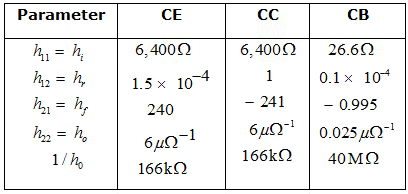Typical h-parameters for A2N404 Germanium Transistor, Evaluated at Ic = 1mA,  VCE = 6V, f = 1kHz, and TA = 25 degree Centrigrade.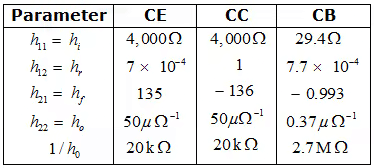• If the h-parameters are given for one type of amplifier connection but are required for another type, the approximate conversion formulae in may be used.

Approximate Conversion Formulae for Transistor h-parameters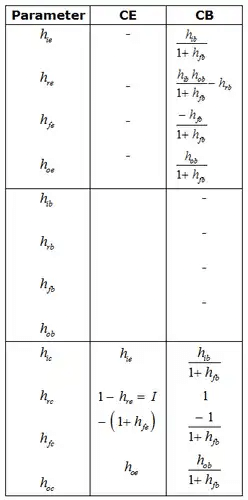• Knowing the h-parameters for a transistor, the values for Ai, Ri, Av, Ro Avg and Aig may be obtained using the exact equations in the table by using a second subscript appropriate to the amplifier being analyzed. The value of Rg and RL are also necessary.
• Exact equations for the small signal analysis of transistor amplifiers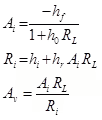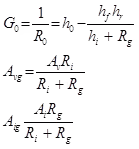Using a transistor’s h-parameters, it is possible to dually match Rg and PL for the Maximum Available Power Gain (MAG) from the transistor, Equations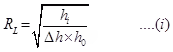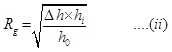• Where Δh= hi h- hhf
Circuit schematic hybrid model and V-I, h-parameter equations for the CE, CC and CB amplifier connections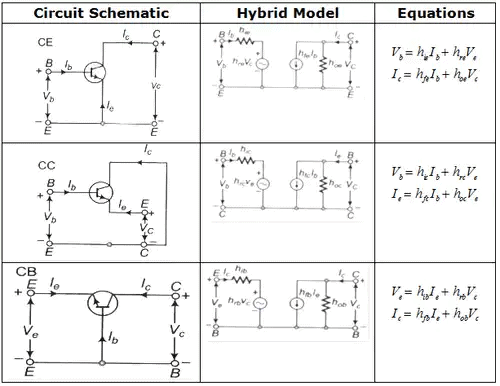• A transistor amplifier’s characteristics are very dependent upon the values used for Rg and RL, but generally speaking, the characteristics may be summarized as in the given ahead.
Comparison between CB, CE, CC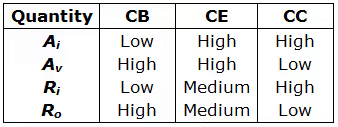Approximate equations for Ai, Ri, Av and R0 in terms of CE h-parameter are shown in table and are valid if, hoeR≤ 0.1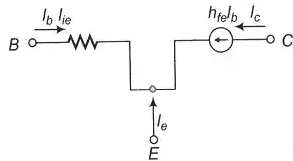Approximate hybrid equivalent circuit at low frequencies, when at low frequencies, when hoeRL ≤ 0.1
• hoe=he=0
• Approximate equations for Ai, Ri, Aand R0 for CB, CE or CC amplifiers, given in terms of Ce h-parameters. To be used only when hoe RL or hoe he<=0.1  is given below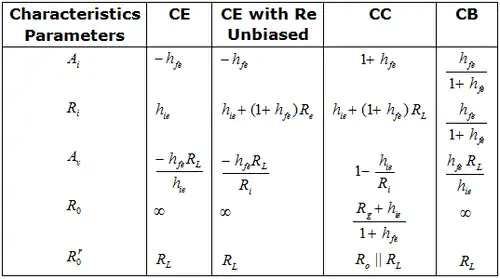➤ Low-Frequency Response of Amplifier

• The value of the emitter bypass capacitor in a single stage CE amplifier must be very large to provide a low value for the lower 3-dB frequency. f1 equations for f1 for different circuit conditions are summarized in the table.
• Summary of the approximate equations for mid frequency voltage gain and lower 3-dB frequency f1. For various circuit conditions: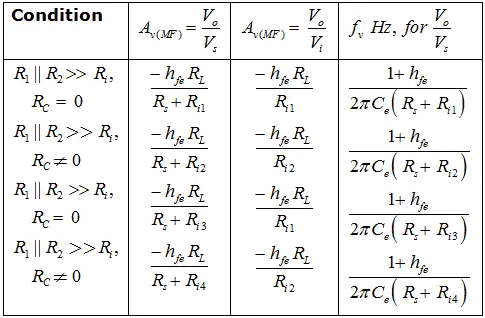• The electrolyte losses in the emitter bypass capacitor affect the low-frequency response some what but have a significant effect on mid frequency gain.
• Low-frequency response is also affected by the size of the coupling capacitors, as shown by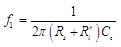Where R'i is the effective input resistance to the amplifier.
• The overall mid frequency voltage gain of an amplifier consisting of cascaded CE stages is the product of the gains of all of the stages. But the overall current gain must take into account the load and be biasing resistors, along with the transistor input impedances.
• Analysis of cascaded stages generally proceeds from the last stage back towards the input, by determining Ai, Ri and Av in that order.
• The overall low-frequency response, f1(n) for n cascaded stages each having the same value of f1 given by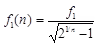which shows that the low-frequency response is poorer than for a single stage.
• The ability of an amplifier to handle a square wave signal is measured by the sag and is related to the amplifiers lower 3-dB frequency,  f1 by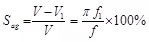where f is the frequency of the square wave. To provide a low Sag requires a very low value for f1.
• Cascaded transformer coupled amplifiers designed for maximum power transfer conditions have equal current and voltage gains given by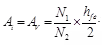• The lower 3-dB frequency f1, for a transformer coupled amplifier, is restricted by the transformer’s primary inductance Lp, as given by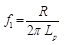Where R is the effective portion of the transistor’s load and output resistance in parallel with Lp.
• In order for a transformer coupled amplifier to handle a pulse of duration td with less than a given amount of Sag, the transformer must have a minimum primary inductance is given by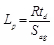➤ High-Frequency Response of Amplifiers

• The hybrid-π model of a transistor at high frequencies includes the capacitive effects of the p-n junction and involves a base spreading resistance that creates a virtual base.
• The transistor’s high-frequency parameters are given in equations below and include the transistors transconductance.
• The parameters for the approximate hybrid-π model may be obtained from the following equations. (Numerical values shown are for 1 2N 2218 silicon transistor with: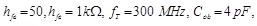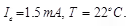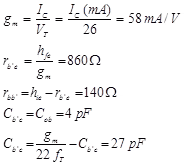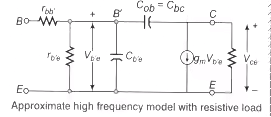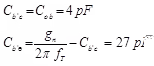Cb’c is usually given in the transistor manual as Cob and is the CB open circuit output capacitance Cb’e may be given the manufacturer or it may be calculated using the equation given, ft is the frequency at which the CE short circuit current gain drops to unity.
• The transistor’s short circuit current gain varies with frequency and is characterized by the α cut-off and β cut off frequencies, where the current gain drops by 3 dB from the value at mid frequencies in a CB and CE connection respectively.
• Important high-frequency characteristics of a transistor are ft, the gain bandwidth product and are defined to be the frequency at which a common emitter’s short circuit current gain drops to unity.
• Cascaded CE stages operating at high frequency have a reduced overall bandwidth given by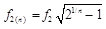where n is the number of stages and f2 is the bandwidth of each stage
• The ability of an amplifier to reproduce a square wave input with a low rise time, tr, is directly proportional to the bandwidth f2. That is
tr = 0.35/f2
• When a transistor is operated at high frequencies with a resistive load, the collector to base junction capacitance appears as an enlarged value of capacitance at the input, thus reducing voltage and current gain below the values expected at the α and β cut-off.
• The high-frequency response of a transformer coupled amplifier is usually limited entirely by the transformer’s leakage inductance and distributed capacitance, which creates a series resonant effect.
• Transistor noise is usually measured as a spot noise figure, NF or as the average noise figure NF, with typical values between 0.5 and 5 dB depending upon the source resistance, collector current, and frequency.
The document Frequency Response Notes | Study Analog Circuits - Electronics and Communication Engineering (ECE) is a part of the Electronics and Communication Engineering (ECE) Course Analog Circuits.
All you need of Electronics and Communication Engineering (ECE) at this link: Electronics and Communication Engineering (ECE)

## Analog Circuits

1 videos|17 docs|43 tests
 Use Code STAYHOME200 and get INR 200 additional OFF

## Analog Circuits

1 videos|17 docs|43 tests

Track your progress, build streaks, highlight & save important lessons and more!

,

,

,

,

,

,

,

,

,

,

,

,

,

,

,

,

,

,

,

,

,

;